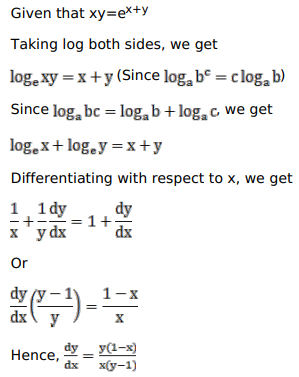# Solve this following

Question:

Mark $(\sqrt{)}$ against the correct answer in the following:

If $e^{x+y}=x y$ then $\frac{d y}{d x}=?$

A. $\frac{x(1-y)}{y(x-1)}$

B. $\frac{y(1-x)}{x(y-1)}$

C. $\frac{(x-x y)}{(x y-y)}$

D. none of these

Solution: